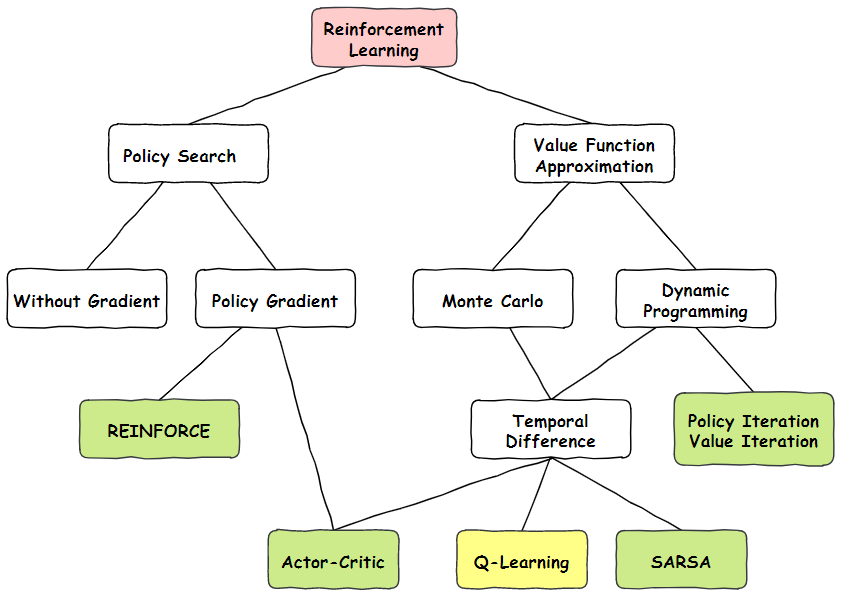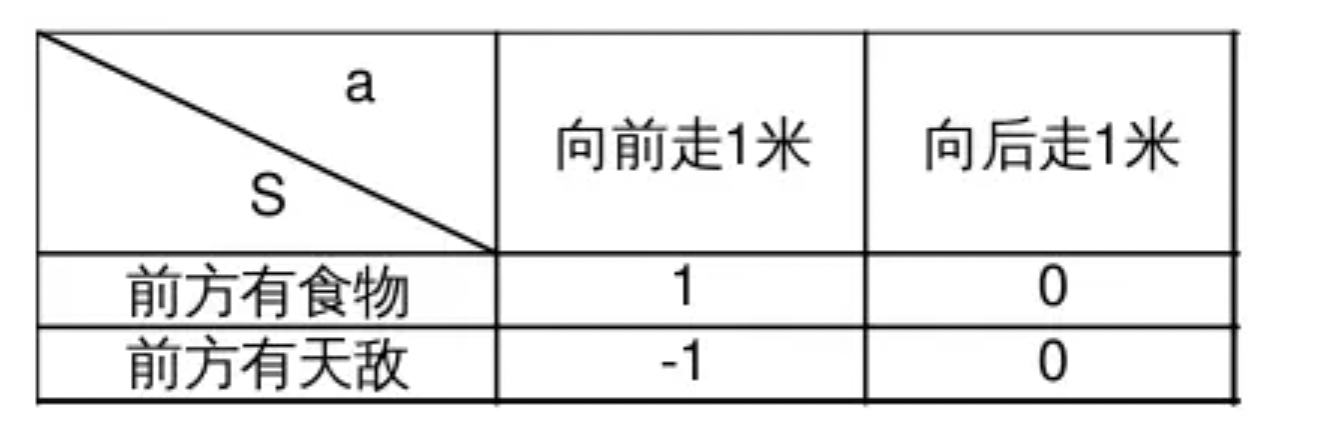# Q-learning¶

## 介绍¶首先我们看一下上图Q-learning在整个强化学习的位置，Q-Learning是属于值函数近似算法中，蒙特卡洛方法和时间差分法相结合的算法。它在1989年被Watkins提出，可以说一出生就给强化学习带来了重要的突破。

Q-Learning假设可能出现的动作a和状态S是有限多，这时a和S的全部组合也是有限多个，并且引入价值量Q表示智能体认为做出某个a时所能够获得的利益。在这种假设下，智能体收到S，应该做出怎样的a，取决于选择哪一个a可以产生最大的Q。下面的表格显示了动物在面对环境的不同状态时做出的a对应着怎样的Q，这里为了简单说明只分别列举了2种S和a：## 基础概念¶

Q-Learning的训练过程是Q表的Q值逐渐调整的过程，其核心是根据已经知道的Q值，当前选择的行动a作用于环境获得的回报R和下一轮$$S_{t+1}$$对应可以获得的最大利益Q，总共三个量进行加权求和算出新的Q值，来更新Q表：

$Q(S_{t},A_{t})=Q(S_{t},A_{t})+\alpha[R_{t+1}+\gamma \mathop{max}_{a} Q(S_{t+1},a)-Q(S_{t},A_{t})]$

## 应用举例¶$Q( (3,2) down) = Q( (3,2) down ) + 0.1× ( -0.4 + 0.5 × max [Q( (4,2) action) ]- Q( (3,2), down））$
$Q( (3,2), down) = 0.6 + 0.1× ( -0.4 + 0.5 × max [0.2, 0.4, 0.6] – 0.6)=0.53$

## 优缺点¶

Q-Learning算法有一些缺点，比如状态和动作都假设是离散且有限的，对于复杂的情况处理起来会很麻烦；智能体的决策只依赖当前环境的状态，所以如果状态之间存在时序关联那么学习的效果就不佳。